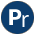#oset.pl -- Ordered set manipulation

This library defines set operations on sets represented as ordered lists. This current library is a thin wrapper around library(ordsets). Many of the implementations of library(ordsets) originate from the library.

author
- Jon Jagger
deprecated
- Use the de-facto library(ordsets)
oset_is(@OSet)
check that OSet in correct format (standard order)
deprecated
- Use is_ordset/1 from library(ordsets)
oset_union(+OSet1, +OSet2, -Union)
Union is the union of OSet1 and OSet2.
deprecated
- Use ord_union/3 from library(ordsets)
oset_int(+OSet1, +OSet2, -Int)
ordered set intersection
oset_diff(+InOSet, +NotInOSet, -Diff)
Ordered set difference
deprecated
- Use ord_subtract/3 from library(ordsets)
oset_dunion(+SetofSets, -DUnion)
Distributed union.
deprecated
- Use ord_union/2 from library(ordsets)
oset_dint(+SetofSets, -DInt)
Distributed intersection.
deprecated
- Use ord_intersection/2 from library(ordsets)
oset_power(+Set, -PSet)
True when PSet is the powerset of Set. That is, Pset is a set of all subsets of Set, where each subset is a proper ordered set.
oset_addel(+Set, +El, -Add)
Ordered set element addition.
deprecated
- Use ord_add_element/3 from library(ordsets)
oset_delel(+Set, +El, -Del)
Ordered set element deletion.
deprecated
- Use ord_del_element/3 from library(ordsets)